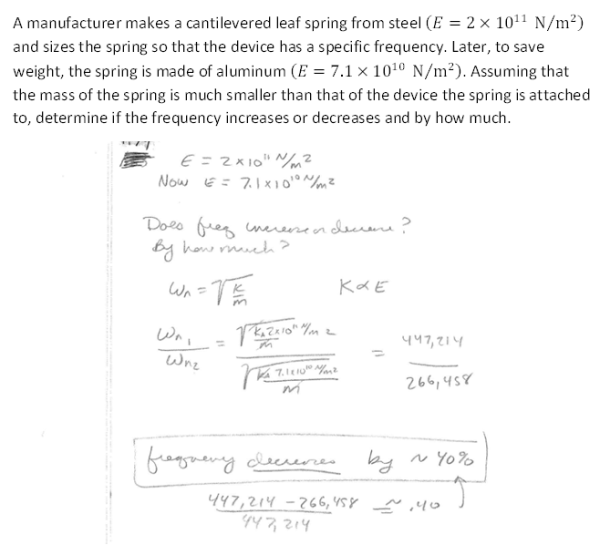A manufacturer makes a cantilevered leaf spring from steel (E= 2x10^11 N/m^2) and sizes the spring so that the decive has a specific frequency. Later, to save weight, the spring is made of aluminum (E=7.1x10^10 N/m^2). Assuming that the mass of the spring is much smaller than that of the device the spring is attached to, determine if the frequency increases or decreases and by how much.A manufacturer makes a cantilevered leaf spring from steel (E= 2x10^11 N/m^2) and sizes the spring so that the decive has a specific frequency. Later, to save weight, the spring is made of aluminum (E=7.1x10^10 N/m^2). Assuming that the mass of the spring is much smaller than that of the device the spring is attached to, determine if the frequency increases or decreases and by how much.

Vibrations Page 1 vibrations vibrations vibrations vibrations vibrations vibrations vibrations Vibrations Page 2